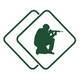#### ASVAB Armed Services Vocational Aptitude Battery (Exam 30)

created by Fisher BRink (@fisher) at Feb. 8, 2016
• Solve for x: -x2 x + 30 = 0

• A square box has a volume of 64 cubic inches. What's its perimeter?

• A cube has a volume of 64 cubic inches.What's its surface area?

• (x3)3 = ?

• 4! =

• If a3 + b3 = a3 + x3, then b =

• Triangle ABC as shown above is a(n):

• The angles of the quadrilateral above:

• In the above figure, the sum of Angles 1 and 2 equals:

• 3(2x 5) 2(4x + 1) = 5(x + 3) 2

• A cube has a volume of 64-cubic inches.What's the length of one side of the cube?

• (x3)2 =

• If I inches of rain fall in one minute, how many inches fall in H hours?

• If x = y, 6 + 4 (x y) =

• A circle measures 12 feet in diameter. What's its area to the nearest foot?

• A square box has 6-inch sides. What's its volume?

• A cylinder has a diameter of 10 inches.What's its approximate area?

• A cylinder has a diameter of 12 inches and a height of 10 inches. What's its appro...

• 820 is a number between:

• (x + 2)(x + 2) =

Be the first to review

• info
Quiz Info
• date_range
Feb. 8, 2016, 3:26 a.m.
help_outline
20 questions
dvr
0 completed
remove_red_eye
14 views
people
0 takers
folder•#### Ratings

star_borderstar_borderstar_borderstar_borderstar_border
ratings